# AutoML之自动化特征工程

## 1. 引言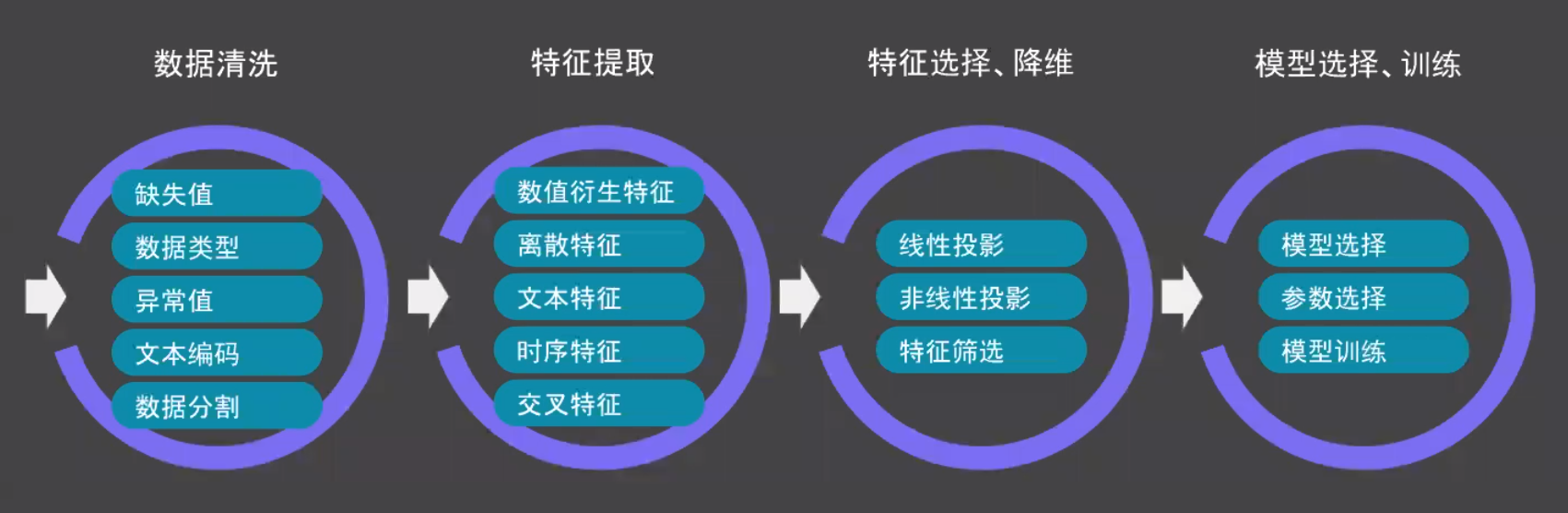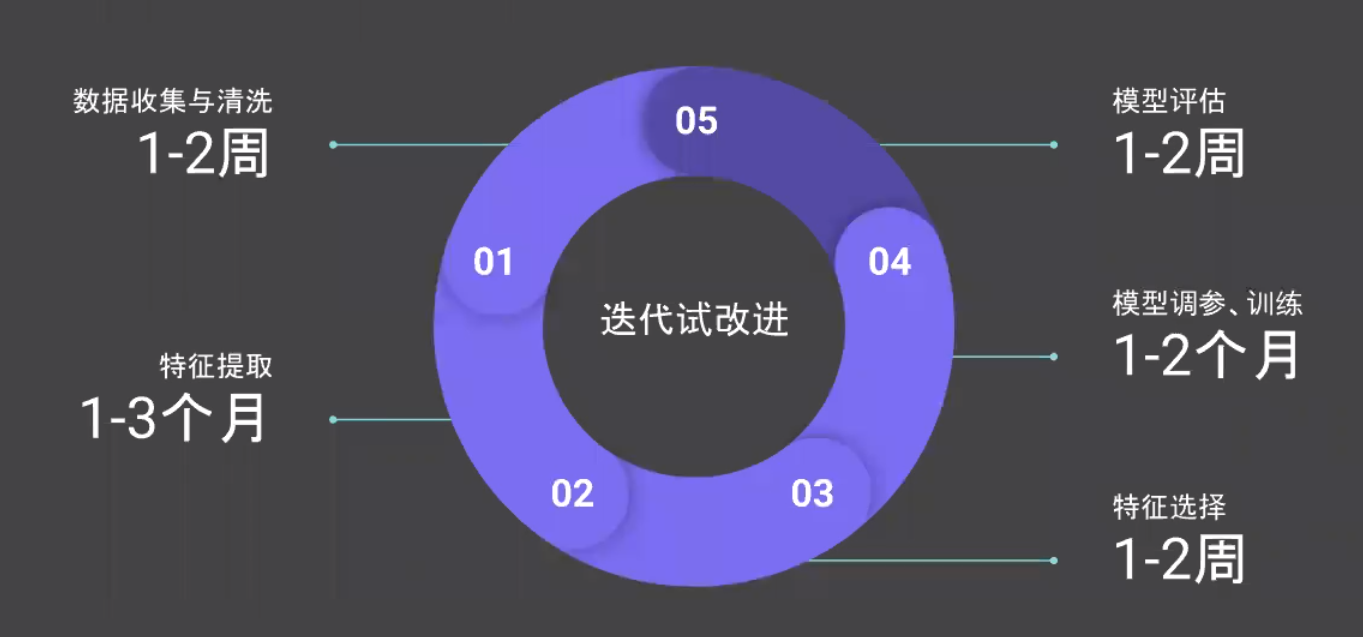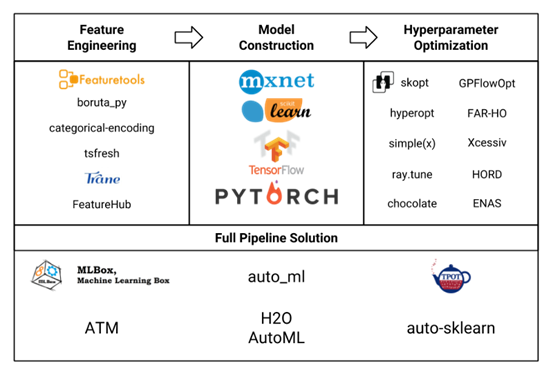## 3. 自动化特征工程工具包

### 3.1 Featuretools

Featuretools使用一种称为深度特征合成（Deep Feature Synthesis，DFS）的算法，该算法遍历通过关系数据库的模式描述的关系路径。当DFS遍历这些路径时，它通过应用于数据的操作（包括和、平均值和计数）生成综合特征。例如，对来自给定字段client_id的事务列表应用sum操作，并将这些事务聚合到一个列中。尽管这是一个深度操作，但该算法可以遍历更深层的特征。Featuretools最大的优点是其可靠性和处理信息泄漏的能力，同时可以用来对时间序列数据进行处理。

clients ：有关信用合作社客户的基本信息表。每个客户端在此数据框中只有一行。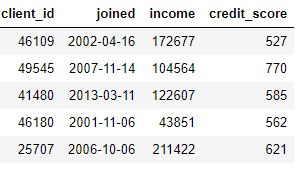loans：向客户提供的贷款表。每笔贷款在此数据框中只有自己的行，但客户可能有多笔贷款。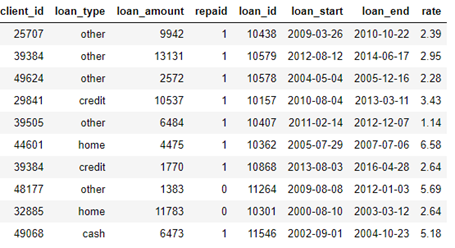payments：贷款偿还表。每笔付款只有一行，但每笔贷款都有多笔付款。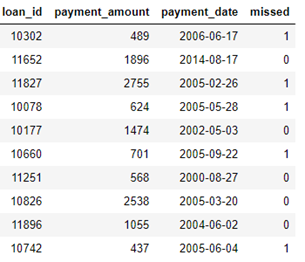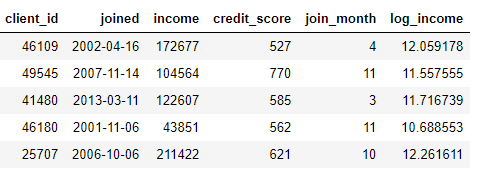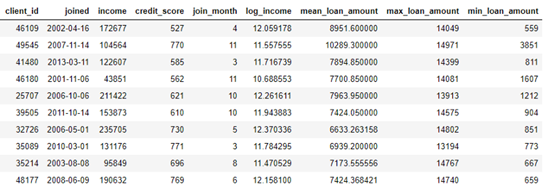Featuretools有两个主要概念：

• 第一个是entities，它可被视为单个表。
• 第二个是entityset，它是实体(表)的集合，以及用来表示实体之间的关系。

import featuretools as ft

es = ft.EntitySet(id='clients')

es = es.entity_from_dataframe(entity_id='clients', dataframe=clients,
index='client_id', time_index='joined')
es = es.entity_from_dataframe(entity_id='loans', dataframe=loans,
index='loans_id', time_index='joined')

es = es.entity_from_dataframe(entity_id='payments', dataframe=payments,
variable_types={'missed': ft.variable_types.Categorical},
make_index=True, index='payment_id', time_index='payment_date')

# 'clients'表与loans表关联
r_client_previous = ft.Relationship(es['clients']['client_id'], es['loans']['client_id'])

# 将关系添加到实体集

# loans表与payments表关联
r_payments = ft.Relationship(es['loans']['loan_id'], es['payments']['loan_id'])

# 将关系添加到实体集
es = es.add_relationship(r_payments)

• Aggregations:分组聚合
• Transformations:列之间计算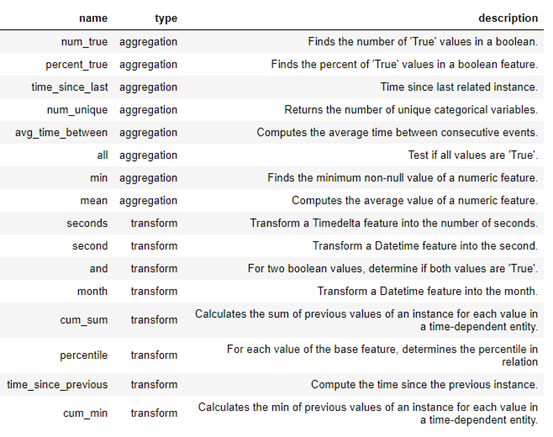features, feature_names = ft.dfs(entityset=es, target_entity='clients',
agg_primitives=['mean', 'max', 'percent_true', 'last'],
trans_primitives=['years', 'month', 'subtract', 'divide'])

features, feature_names = ft.dfs(entityset=es, target_entity='clients', max_depth=2)

### 3.2 Boruta

Boruta主要是用来进行特征选择。所以严格意义上，Boruta并不是我们所需要的自动化特征工程包。

Boruta-py是brouta特征约简策略的一种实现，在该策略中，问题以一种完全相关的方式构建，算法保留对模型有显著贡献的所有特征。这与许多特征约简算法所应用的最小最优特征集相反。boruta方法通过创建由目标特征的随机重排序值组成的合成特征来确定特征的重要性，然后在原始特征集的基础上训练一个简单的基于树的分类器，在这个分类器中，目标特征被合成特征所替代。所有特性的性能差异用于计算相对重要性。

Boruta函数通过循环的方式评价各变量的重要性，在每一轮迭代中，对原始变量和影子变量进行重要性比较。如果原始变量的重要性显著高于影子变量的重要性，则认为该原始变量是重要的；如果原始变量的重要性明显低于影子变量的重要性，则认为该原始变量是不重要的。其中，原始变量就是我们输入的要进行特征选择的变量；影子变量就是根据原始变量生成的变量

• 先向原始变量中加入随机干扰项，这样得到的是扩展后的变量
• 从扩展后的变量中进行抽样，得到影子变量

# 从训练数据集获取特征
z = train_df[f].values

# Shuffle
np.random.shuffle(z)

# 影子特征
train_df[f + "shadow"] = z

1. 首先，它通过创建混合数据的所有特征（即影子特征）为给定的数据集增加了随机性。
2. 然后，它训练一个随机森林分类的扩展数据集，并采用一个特征重要性措施（默认设定为平均减少精度），以评估的每个特征的重要性，越高则意味着越重要。
3. 在每次迭代中，它检查一个真实特征是否比最好的影子特征具有更高的重要性（即该特征是否比最大的影子特征得分更高）并且不断删除它视为非常不重要的特征。
4. 最后，当所有特征得到确认或拒绝，或算法达到随机森林运行的一个规定的限制时，算法停止。

### 3.3 tsfresh

tsfresh是基于可伸缩假设检验的时间序列特征提取工具。该包包含多种特征提取方法和鲁棒特征选择算法。

tsfresh可以自动地从时间序列中提取100多个特征。这些特征描述了时间序列的基本特征，如峰值数量、平均值或最大值，或更复杂的特征，如时间反转对称性统计量等。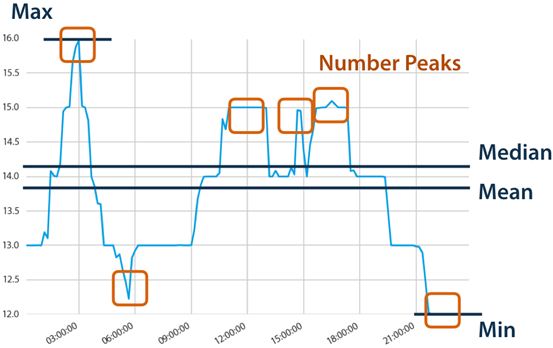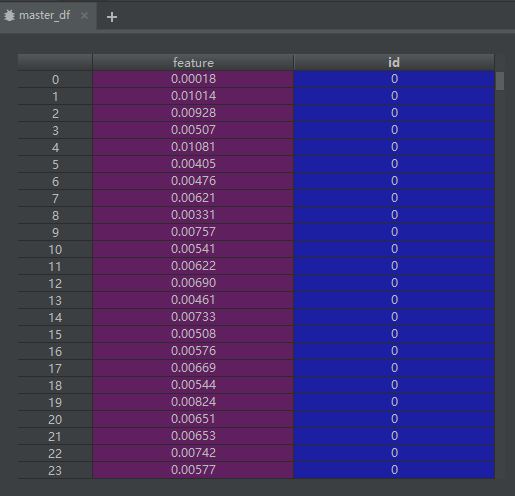import matplotlib.pylab as plt
from tsfresh import extract_features, select_features
from tsfresh.utilities.dataframe_functions import impute
from tsfresh.feature_extraction import ComprehensiveFCParameters
from sklearn.tree import DecisionTreeClassifier
from sklearn.model_selection import train_test_split
from sklearn.metrics import classification_report
import pandas as pd
import numpy as np

if __name__ == '__main__':
N = 500

# plt.plot(df.ix[0, :])
# plt.show()

#
extraction_settings = ComprehensiveFCParameters()
master_df = pd.DataFrame({'feature': df[:N].values.flatten(),
'id': np.arange(N).repeat(df.shape)})

# 时间序列特征工程
X = extract_features(timeseries_container=master_df, n_jobs=0, column_id='id', impute_function=impute,
default_fc_parameters=extraction_settings)

X_train, X_test, y_train, y_test = train_test_split(X, y, test_size=0.2)
cl = DecisionTreeClassifier()
cl.fit(X_train, y_train)
print(classification_report(y_test, cl.predict(X_test)))

# 未进行时间序列特征工程
X_1 = df.ix[:N - 1, :]
X_train, X_test, y_train, y_test = train_test_split(X_1, y, test_size=.2)
cl = DecisionTreeClassifier()
cl.fit(X_train, y_train)
print(classification_report(y_test, cl.predict(X_test)))

relevant_features = set()
for label in y.unique():
y_train_binary = y_train == label
X_train_filtered = select_features(X_train, y_train_binary)
print("Number of relevant features for class {}: {}/{}".format(label, X_train_filtered.shape,
X_train.shape))
relevant_features = relevant_features.union(set(X_train_filtered.columns))
X_train_filtered = X_train[list(relevant_features)]
X_test_filtered = X_test[list(relevant_features)]
cl = DecisionTreeClassifier()
cl.fit(X_train_filtered, y_train)
print(classification_report(y_test, cl.predict(X_test_filtered)))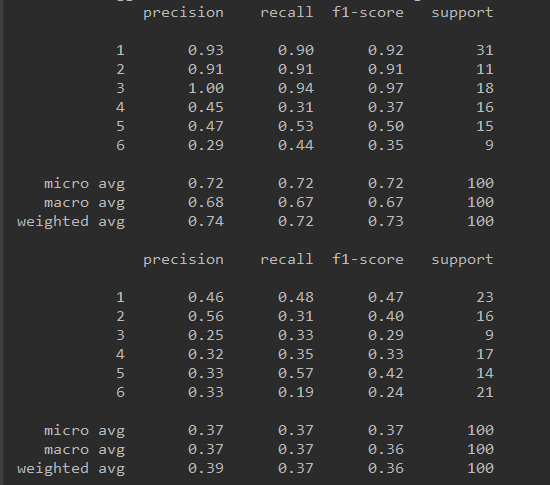## 4. 总结

posted @ 2019-02-14 22:12  Bo_hemian  阅读(11215)  评论(1编辑  收藏  举报